xElementary chargeEncyclopedia
The elementary charge, usually denoted as e, is the electric charge
Electric charge
Electric charge is a physical property of matter that causes it to experience a force when near other electrically charged matter. Electric charge comes in two types, called positive and negative. Two positively charged substances, or objects, experience a mutual repulsive force, as do two...

carried by a single proton
Proton
The proton is a subatomic particle with the symbol or and a positive electric charge of 1 elementary charge. One or more protons are present in the nucleus of each atom, along with neutrons. The number of protons in each atom is its atomic number....

, or equivalently, the absolute value
Absolute value
In mathematics, the absolute value |a| of a real number a is the numerical value of a without regard to its sign. So, for example, the absolute value of 3 is 3, and the absolute value of -3 is also 3...

of the electric charge carried by a single electron
Electron
The electron is a subatomic particle with a negative elementary electric charge. It has no known components or substructure; in other words, it is generally thought to be an elementary particle. An electron has a mass that is approximately 1/1836 that of the proton...

. This elementary charge is a fundamental physical constant
Physical constant
A physical constant is a physical quantity that is generally believed to be both universal in nature and constant in time. It can be contrasted with a mathematical constant, which is a fixed numerical value but does not directly involve any physical measurement.There are many physical constants in...

. To avoid confusion over its sign, e is sometimes called the elementary positive charge. This charge has a measured value of approximately In the cgs system, e is .

## Elementary charge as a unit

In some natural unit systems, such as the system of atomic units
Atomic units
Atomic units form a system of natural units which is especially convenient for atomic physics calculations. There are two different kinds of atomic units, which one might name Hartree atomic units and Rydberg atomic units, which differ in the choice of the unit of mass and charge. This article...

, e functions as the unit
Units of measurement
A unit of measurement is a definite magnitude of a physical quantity, defined and adopted by convention and/or by law, that is used as a standard for measurement of the same physical quantity. Any other value of the physical quantity can be expressed as a simple multiple of the unit of...

of electric charge, that is e is equal to 1 e in those unit systems. The use of elementary charge as a unit was promoted by George Johnstone Stoney
George Johnstone Stoney
George Johnstone Stoney was an Irish physicist most famous for introducing the term electron as the "fundamental unit quantity of electricity"....

in 1874 for the first system of natural units
Natural units
In physics, natural units are physical units of measurement based only on universal physical constants. For example the elementary charge e is a natural unit of electric charge, or the speed of light c is a natural unit of speed...

, called Stoney units. Later, he proposed the name electron for this unit. At the time, the particle we now call the electron
Electron
The electron is a subatomic particle with a negative elementary electric charge. It has no known components or substructure; in other words, it is generally thought to be an elementary particle. An electron has a mass that is approximately 1/1836 that of the proton...

was not yet discovered and the difference between the particle electron and the unit of charge electron was still blurred. Later, the name electron was assigned to the particle and the unit of charge e lost its name. However, the unit of energy electronvolt
Electronvolt
In physics, the electron volt is a unit of energy equal to approximately joule . By definition, it is equal to the amount of kinetic energy gained by a single unbound electron when it accelerates through an electric potential difference of one volt...

reminds us that the elementary charge was once called electron.

The magnitude of the elementary charge was first measured in Robert A. Millikan's noted oil drop experiment in 1909.

## Quantization

Charge quantization
Quantization (physics)
In physics, quantization is the process of explaining a classical understanding of physical phenomena in terms of a newer understanding known as "quantum mechanics". It is a procedure for constructing a quantum field theory starting from a classical field theory. This is a generalization of the...

is the concept that every stable and independent object (meaning an object that can exist independently for a prolonged period of time) has a charge that is an integer
Integer
The integers are formed by the natural numbers together with the negatives of the non-zero natural numbers .They are known as Positive and Negative Integers respectively...

multiple of the elementary charge e. Thus, e.g., a charge can be exactly 0 e, or exactly 1 e, −1 e, 2 e, etc., but not, say,  e, or −3.8 e, etc. (This statement must not be interpreted to include quark
Quark
A quark is an elementary particle and a fundamental constituent of matter. Quarks combine to form composite particles called hadrons, the most stable of which are protons and neutrons, the components of atomic nuclei. Due to a phenomenon known as color confinement, quarks are never directly...

s or quasiparticle
Quasiparticle
In physics, quasiparticles are emergent phenomena that occur when a microscopically complicated system such as a solid behaves as if it contained different weakly interacting particles in free space...

s, since neither quarks nor quasiparticles possess the ability to exist on their own for prolonged periods of time. Quarks have charges that are integer multiples of  e.)

This is the reason for the terminology "elementary charge": it is meant to imply that it is an indivisible unit of charge.

### Charges less than an elementary charge

There are two known sorts of exceptions to the indivisibility of the elementary charge: quark
Quark
A quark is an elementary particle and a fundamental constituent of matter. Quarks combine to form composite particles called hadrons, the most stable of which are protons and neutrons, the components of atomic nuclei. Due to a phenomenon known as color confinement, quarks are never directly...

s and quasiparticle
Quasiparticle
In physics, quasiparticles are emergent phenomena that occur when a microscopically complicated system such as a solid behaves as if it contained different weakly interacting particles in free space...

s.
• Quark
Quark
A quark is an elementary particle and a fundamental constituent of matter. Quarks combine to form composite particles called hadrons, the most stable of which are protons and neutrons, the components of atomic nuclei. Due to a phenomenon known as color confinement, quarks are never directly...

s, first posited in the 1960s, have quantized charge, but the charge is quantized into multiples of  e. However, quarks cannot be seen as isolated particles; they exist only in groupings, and stable groupings of quarks (such as a proton
Proton
The proton is a subatomic particle with the symbol or and a positive electric charge of 1 elementary charge. One or more protons are present in the nucleus of each atom, along with neutrons. The number of protons in each atom is its atomic number....

, which consists of three quarks) all have charges that are integer multiples of e. For this reason, either 1 e or  e can be justifiably considered to be "the quantum
Quantum
In physics, a quantum is the minimum amount of any physical entity involved in an interaction. Behind this, one finds the fundamental notion that a physical property may be "quantized," referred to as "the hypothesis of quantization". This means that the magnitude can take on only certain discrete...

of charge", depending on the context.

• Quasiparticle
Quasiparticle
In physics, quasiparticles are emergent phenomena that occur when a microscopically complicated system such as a solid behaves as if it contained different weakly interacting particles in free space...

s are not particles as such, but rather an emergent
Emergence
In philosophy, systems theory, science, and art, emergence is the way complex systems and patterns arise out of a multiplicity of relatively simple interactions. Emergence is central to the theories of integrative levels and of complex systems....

entity in a complex material system that behaves like a particle. In 1982 Robert Laughlin
Robert B. Laughlin
Robert Betts Laughlin is a professor of Physics and Applied Physics at Stanford University. Along with Horst L. Störmer of Columbia University and Daniel C. Tsui of Princeton University, he was awarded a share of the 1998 Nobel Prize in physics for their explanation of the fractional quantum Hall...

explained the fractional quantum Hall effect
Fractional quantum Hall effect
The fractional quantum Hall effect is a physical phenomenon in which the Hall conductance of 2D electrons shows precisely quantised plateaus at fractional values of e^2/h. It is a property of a collective state in which electrons bind magnetic flux lines to make new quasiparticles, and excitations...

by postulating the existence of fractionally-charged quasiparticle
Quasiparticle
In physics, quasiparticles are emergent phenomena that occur when a microscopically complicated system such as a solid behaves as if it contained different weakly interacting particles in free space...

s. This theory is now widely accepted, but this is not considered to be a violation of the principle of charge quantization, since quasiparticles are not elementary particles.

### What is the quantum of charge?

All known elementary particle
Elementary particle
In particle physics, an elementary particle or fundamental particle is a particle not known to have substructure; that is, it is not known to be made up of smaller particles. If an elementary particle truly has no substructure, then it is one of the basic building blocks of the universe from which...

s, including quarks, have charges that are integer multiples of  e. Therefore, one can say that the "quantum
Quantum
In physics, a quantum is the minimum amount of any physical entity involved in an interaction. Behind this, one finds the fundamental notion that a physical property may be "quantized," referred to as "the hypothesis of quantization". This means that the magnitude can take on only certain discrete...

of charge" is  e. In this case, one says that the "elementary charge" is three times as large as the "quantum of charge".

On the other hand, all isolatable particles have charges that are integer multiples of e. (Quarks cannot be isolated, except in combinations like protons that have total charges that are integer multiples of e.) Therefore, one can say that the "quantum of charge" is e, with the proviso that quarks are not to be included. In this case, "elementary charge" would be synonymous with the "quantum of charge".

In fact, both terminologies are used. For this reason, phrases like "the quantum of charge" or "the indivisible unit of charge" can be ambiguous, unless further specification is given. On the other hand, the term "elementary charge" is unambiguous: It universally refers to the charge of a proton.

If the Avogadro constant NA and the Faraday constant F are independently known, the value of the elementary charge can be deduced, using the formula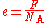(In other words, the charge of one mole of electrons, divided by the number of electrons in a mole, equals the charge of a single electron.)

In practice, this method is not how the most accurate values are measured today: Nevertheless, it is a legitimate and still quite accurate method, and experimental methodologies are described below:

The value of the Avogadro constant NA was first approximated by Johann Josef Loschmidt
Johann Josef Loschmidt
Jan or Johann Josef Loschmidt , who referred to himself mostly as 'Josef' , was a notable Austrian scientist who performed groundbreaking work in chemistry, physics , and crystal forms.Born in Carlsbad, a town located in the Austrian Empire , Loschmidt...

who, in 1865, estimated the average diameter of the molecules in air by a method that is equivalent to calculating the number of particles in a given volume of gas. Today the value of NA can be measured at very high accuracy by taking an extremely pure crystal (in practice, often silicon
Silicon
Silicon is a chemical element with the symbol Si and atomic number 14. A tetravalent metalloid, it is less reactive than its chemical analog carbon, the nonmetal directly above it in the periodic table, but more reactive than germanium, the metalloid directly below it in the table...

), measuring how far apart the atoms are spaced using X-ray diffraction or another method, and accurately measuring the density of the crystal. From this information, one can deduce the mass (m) of a single atom; and since the molar mass
Molar mass
Molar mass, symbol M, is a physical property of a given substance , namely its mass per amount of substance. The base SI unit for mass is the kilogram and that for amount of substance is the mole. Thus, the derived unit for molar mass is kg/mol...

(M) is known, the number of atoms in a mole can be calculated: NA = M/m.

The value of F can be measured directly using Faraday's laws of electrolysis
Faraday's laws of electrolysis are quantitative relationships based on the electrochemical researches published by Michael Faraday in 1834.-Statements of the laws:Several versions of the laws can be found in textbooks and the scientific literature...

Michael Faraday, FRS was an English chemist and physicist who contributed to the fields of electromagnetism and electrochemistry....

in 1834. In an electrolysis
Electrolysis
In chemistry and manufacturing, electrolysis is a method of using a direct electric current to drive an otherwise non-spontaneous chemical reaction...

experiment, there is a one-to-one correspondence between the electrons passing through the anode-to-cathode wire and the ions that plate onto or off of the anode or cathode. Measuring the mass change of the anode or cathode, and the total charge passing through the wire (which can be measured as the time-integral of electric current
Electric current
Electric current is a flow of electric charge through a medium.This charge is typically carried by moving electrons in a conductor such as wire...

), and also taking into account the molar mass of the ions, one can deduce F.

The limit to the precision of the method is the measurement of F: the best experimental value has a relative uncertainty of 1.6 ppm, about thirty times higher than other modern methods of measuring or calculating the elementary charge.

### Oil-drop experiment

A famous method for measuring e is Millikan's oil-drop experiment. A small drop of oil in an electric field would move at a rate that balanced the forces of gravity, viscosity
Viscosity
Viscosity is a measure of the resistance of a fluid which is being deformed by either shear or tensile stress. In everyday terms , viscosity is "thickness" or "internal friction". Thus, water is "thin", having a lower viscosity, while honey is "thick", having a higher viscosity...

(of traveling through the air), and electric force. The forces due to gravity and viscosity could be calculated based on the size and velocity of the oil drop, so electric force could be deduced. Since electric force, in turn, is the product of the electric charge and the known electric field, the electric charge of the oil drop could be accurately computed. By measuring the charges of many different oil drops, it can be seen that the charges are all integer multiples of a single small charge, namely e.

### Shot noise

Any electric current
Electric current
Electric current is a flow of electric charge through a medium.This charge is typically carried by moving electrons in a conductor such as wire...

will be associated with noise
Electronic noise
Electronic noise is a random fluctuation in an electrical signal, a characteristic of all electronic circuits. Noise generated by electronic devices varies greatly, as it can be produced by several different effects...

from a variety of sources, one of which is shot noise
Shot noise
Shot noise is a type of electronic noise that may be dominant when the finite number of particles that carry energy is sufficiently small so that uncertainties due to the Poisson distribution, which describes the occurrence of independent random events, are of significance...

. Shot noise exists because a current is not a smooth continual flow; instead, a current is made up of discrete electrons that pass by one at a time. By carefully analyzing the noise of a current, the charge of an electron can be calculated. This method, first proposed by Walter H. Schottky
Walter H. Schottky
Walter Hermann Schottky was a German physicist who played a major early role in developing the theory of electron and ion emission phenomena, invented the screen-grid vacuum tube in 1915 and the pentode in 1919 while working at Siemens, and later made many significant contributions in the areas of...

, can give only a value of e accurate to a few percent. However, it was used in the first direct observation of Laughlin quasiparticles, implicated in the fractional quantum Hall effect
Fractional quantum Hall effect
The fractional quantum Hall effect is a physical phenomenon in which the Hall conductance of 2D electrons shows precisely quantised plateaus at fractional values of e^2/h. It is a property of a collective state in which electrons bind magnetic flux lines to make new quasiparticles, and excitations...

.

### From the Josephson and von Klitzing constants

Another accurate method for measuring the elementary charge is by inferring it from measurements of two effects in quantum mechanics
Quantum mechanics
Quantum mechanics, also known as quantum physics or quantum theory, is a branch of physics providing a mathematical description of much of the dual particle-like and wave-like behavior and interactions of energy and matter. It departs from classical mechanics primarily at the atomic and subatomic...

: The Josephson effect
Josephson effect
The Josephson effect is the phenomenon of supercurrent across two superconductors coupled by a weak link...

, voltage oscillations that arise in certain superconducting structures; and the quantum Hall effect, a quantum effect of electrons at low temperatures, strong magnetic fields, and confinement into two dimensions. The Josephson constant is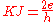(where h is the Planck constant
Planck constant
The Planck constant , also called Planck's constant, is a physical constant reflecting the sizes of energy quanta in quantum mechanics. It is named after Max Planck, one of the founders of quantum theory, who discovered it in 1899...

). It can be measured directly using the Josephson effect
Josephson effect
The Josephson effect is the phenomenon of supercurrent across two superconductors coupled by a weak link...

.

The von Klitzing constant is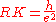It can be measured directly using the quantum Hall effect.

From these two constants, the elementary charge can be deduced: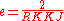### CODATA method

In the most recent CODATA adjustments, the elementary charge is not an independently defined quantity. Instead, a value is derived from the relation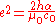where h is the Planck constant
Planck constant
The Planck constant , also called Planck's constant, is a physical constant reflecting the sizes of energy quanta in quantum mechanics. It is named after Max Planck, one of the founders of quantum theory, who discovered it in 1899...

, α is the fine structure constant, μ0 is the magnetic constant and c0 is the speed of light
Speed of light
The speed of light in vacuum, usually denoted by c, is a physical constant important in many areas of physics. Its value is 299,792,458 metres per second, a figure that is exact since the length of the metre is defined from this constant and the international standard for time...

. The uncertainty
Measurement uncertainty
In metrology, measurement uncertainty is a non-negative parameter characterizing the dispersion of the values attributed to a measured quantity. The uncertainty has a probabilistic basis and reflects incomplete knowledge of the quantity. All measurements are subject to uncertainty and a measured...

in the value of e is currently determined entirely by the uncertainty in the Planck constant.

The most precise values of the Planck constant come from watt balance
Watt balance
The watt balance is an experimental electromechanical weight measuring instrument that measures the weight of a test object very precisely by the strength of an electric current and a voltage. It is being developed as a metrological instrument that may one day provide a definition of the kilogram...

experiments, which are currently used to measure the product KRK. The most precise values of the fine structure constant come from comparisons of the measured and calculated value of the gyromagnetic ratio of the electron.